# ARRAYS Variable Arrays Control Arrays Dynamic Variable Arrays

• Slides: 81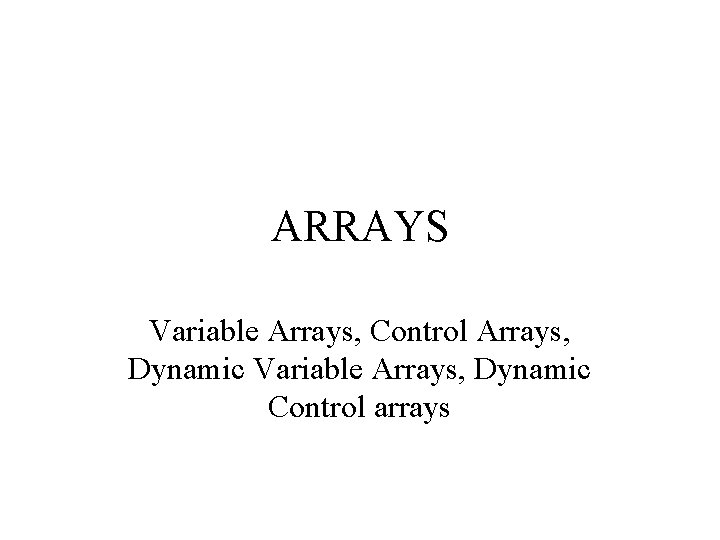ARRAYS Variable Arrays, Control Arrays, Dynamic Variable Arrays, Dynamic Control arrays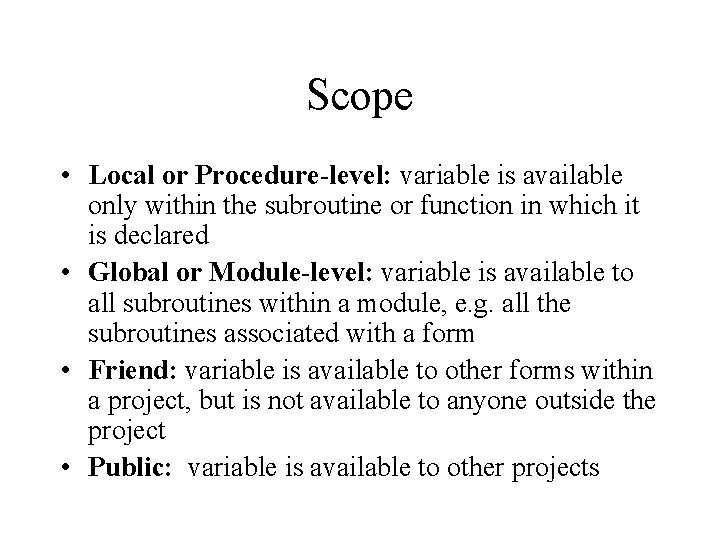Scope • Local or Procedure-level: variable is available only within the subroutine or function in which it is declared • Global or Module-level: variable is available to all subroutines within a module, e. g. all the subroutines associated with a form • Friend: variable is available to other forms within a project, but is not available to anyone outside the project • Public: variable is available to other projectsMulti-Form Demo A Public variable on first form is available to use on the second form.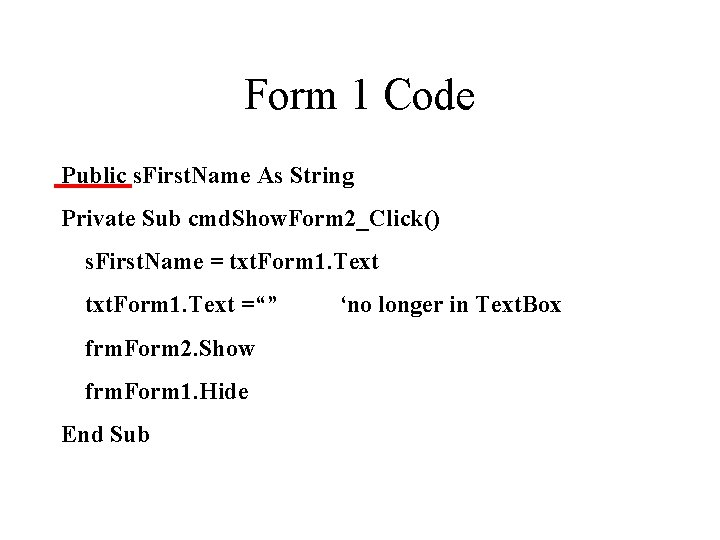Form 1 Code Public s. First. Name As String Private Sub cmd. Show. Form 2_Click() s. First. Name = txt. Form 1. Text =“” frm. Form 2. Show frm. Form 1. Hide End Sub ‘no longer in Text. Box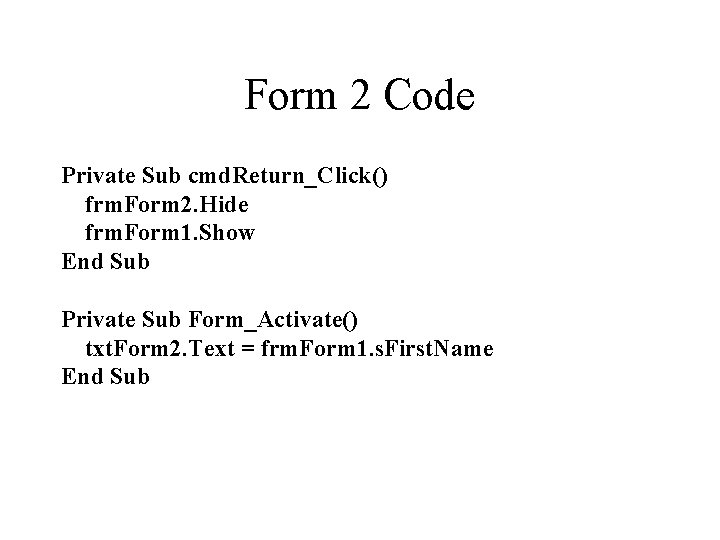Form 2 Code Private Sub cmd. Return_Click() frm. Form 2. Hide frm. Form 1. Show End Sub Private Sub Form_Activate() txt. Form 2. Text = frm. Form 1. s. First. Name End SubVariable Arrays • If a group of variables are – of the same type (e. g. all Integer) • Note: same type does not mean same value. – and play a similar role (i. e. the code for them is nearly identical) it is convenient to make them an array • For example, grade 1, grade 2, … becomes grade(0), grade(1), …. • Chapter 7 in Schneider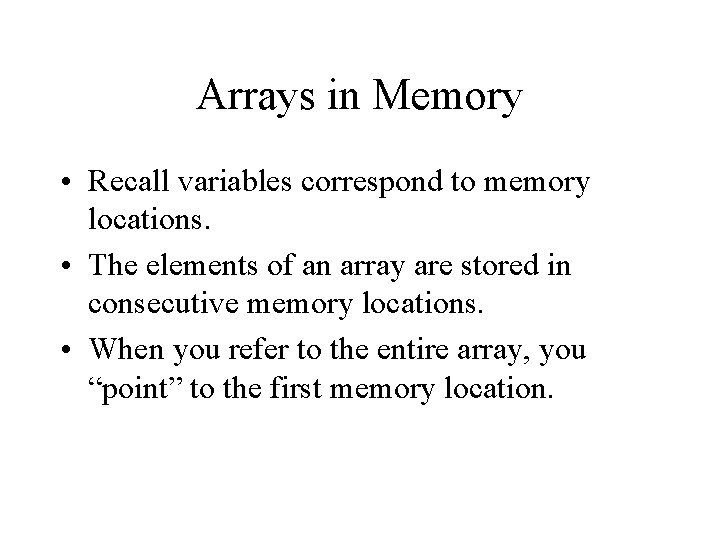Arrays in Memory • Recall variables correspond to memory locations. • The elements of an array are stored in consecutive memory locations. • When you refer to the entire array, you “point” to the first memory location.Before and After Arrays Dim i. Grade 1 As Integer Dim i. Grade 2 As Integer Dim i. Grade 3 As Integer Dim i. Grade 4 As Integer Dim i. Grade 5 As Integer Dim i. Grade 6 As Integer BECOMES Dim i. Grade(5) As Integer ‘zero-basedBefore and After Arrays d. Average = (i. Grade 1 + i. Grade 2 + i. Grade 3 + _ i. Grade 4 + i. Grade 5 + i. Grade 6) / 6 BECOMES d. Average = 0 For i = 0 To 5 d. Average = d. Average + i. Grade(i) Next i d. Average = d. Average / 6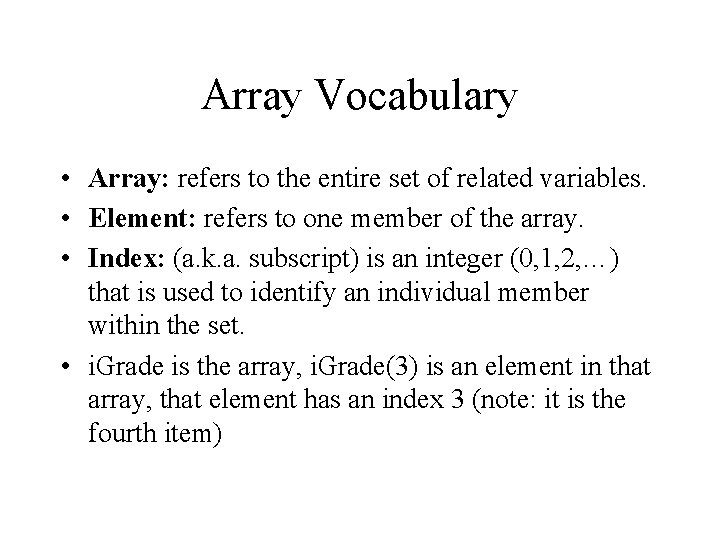Array Vocabulary • Array: refers to the entire set of related variables. • Element: refers to one member of the array. • Index: (a. k. a. subscript) is an integer (0, 1, 2, …) that is used to identify an individual member within the set. • i. Grade is the array, i. Grade(3) is an element in that array, that element has an index 3 (note: it is the fourth item)More Array Vocabulary • Option Base: – If you declare i. Grade(5), VB makes an array with six elements i. Grade(0) through i. Grade(5), that is, it starts indexing at 0. – If you want to start indexing at 1, you can type Option Base 1 (just below Option Explicit). – Note: Control Arrays are always zero based (regardless of Option Base).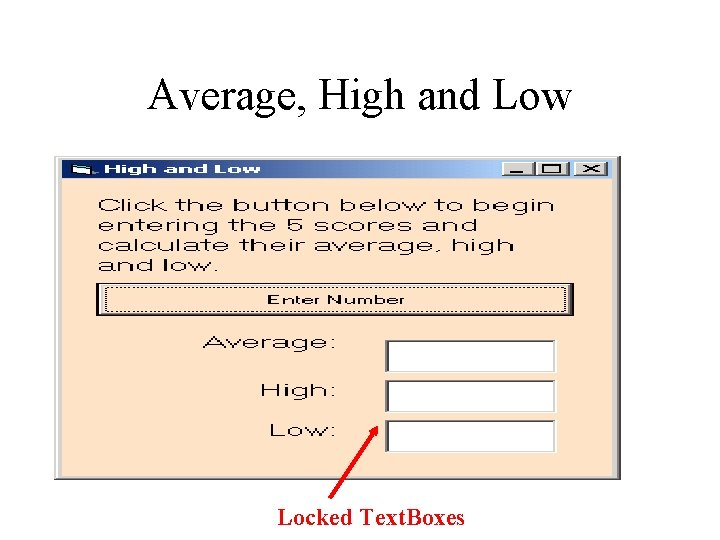Average, High and Low Locked Text. BoxesAverage, High and Low Input Box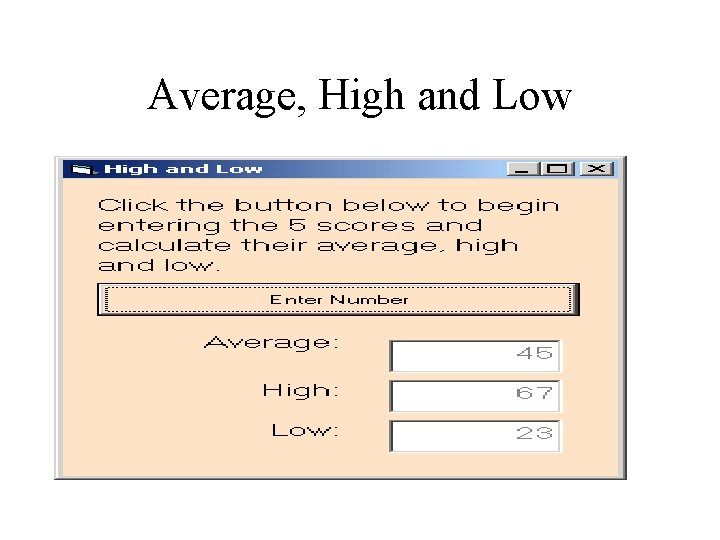Average, High and Low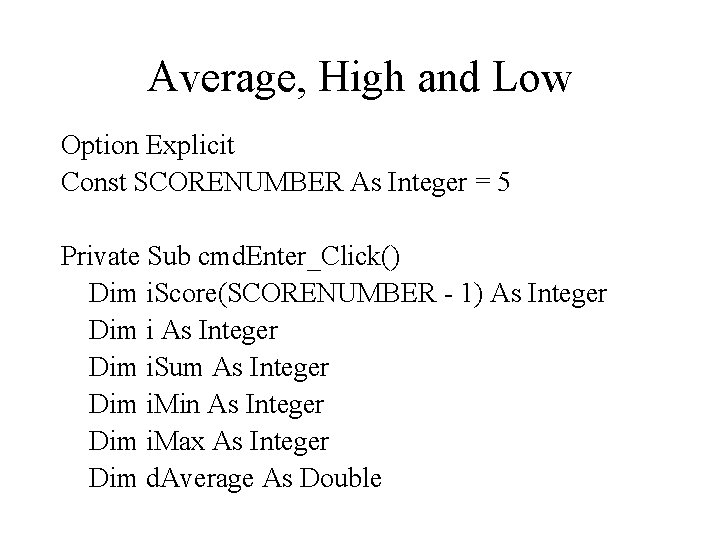Average, High and Low Option Explicit Const SCORENUMBER As Integer = 5 Private Sub cmd. Enter_Click() Dim i. Score(SCORENUMBER - 1) As Integer Dim i. Sum As Integer Dim i. Min As Integer Dim i. Max As Integer Dim d. Average As DoubleAverage, High and Low i. Score(0) = Input. Box("Enter Score 1: ") i. Sum = i. Score(0) i. Min = i. Score(0) i. Max = i. Score(0) ‘Enter first score outside of loop and make it the ‘min and the max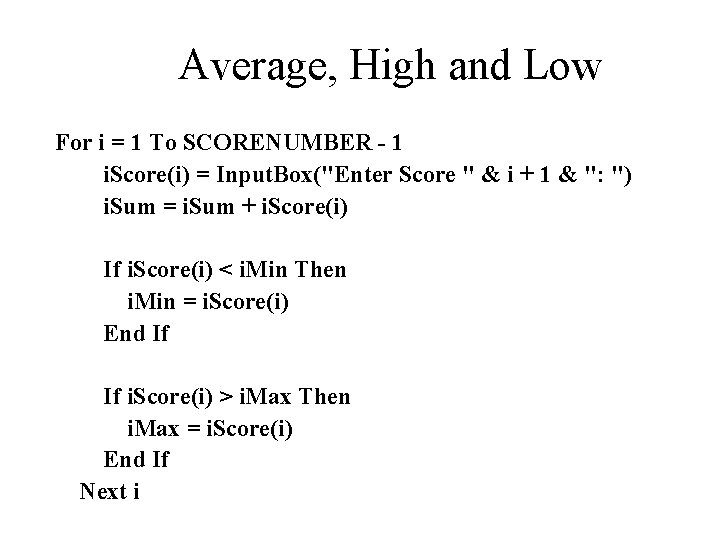Average, High and Low For i = 1 To SCORENUMBER - 1 i. Score(i) = Input. Box("Enter Score " & i + 1 & ": ") i. Sum = i. Sum + i. Score(i) If i. Score(i) < i. Min Then i. Min = i. Score(i) End If If i. Score(i) > i. Max Then i. Max = i. Score(i) End If Next iAverage, High and Low d. Average = Sum / SCORENUMBER txt. Average. Text = d. Average txt. High. Text = i. Max txt. Low. Text = i. Min End Sub Private Sub Form_Load() lbl. Instruct. Caption = "Click the button below to begin " & _ "entering the " & SCORENUMBER & " scores and “ & _ “calculate " & “their average, high and low. " End Sub ‘The use of SCORENUMBER makes the program scale.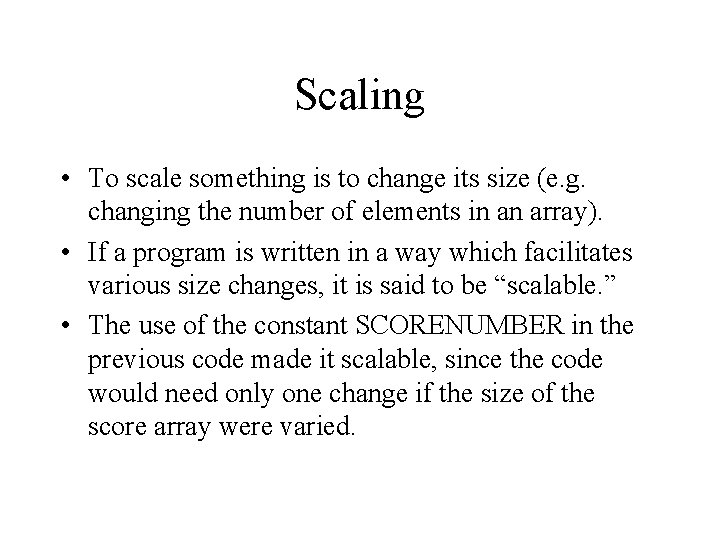Scaling • To scale something is to change its size (e. g. changing the number of elements in an array). • If a program is written in a way which facilitates various size changes, it is said to be “scalable. ” • The use of the constant SCORENUMBER in the previous code made it scalable, since the code would need only one change if the size of the score array were varied.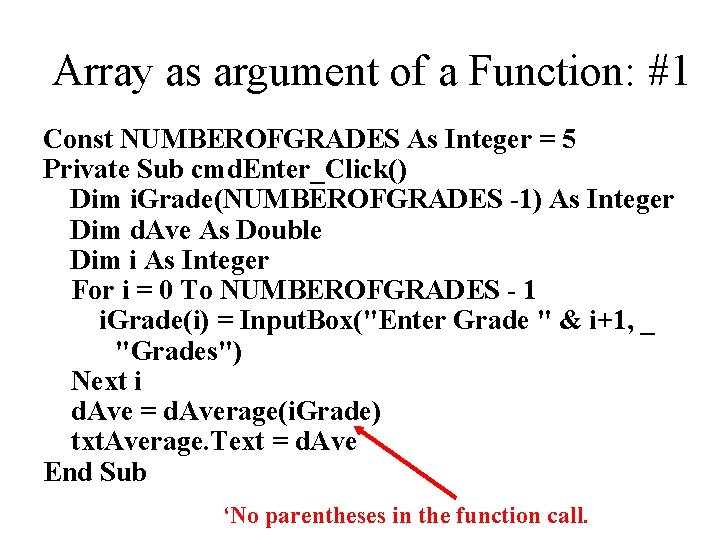Array as argument of a Function: #1 Const NUMBEROFGRADES As Integer = 5 Private Sub cmd. Enter_Click() Dim i. Grade(NUMBEROFGRADES -1) As Integer Dim d. Ave As Double Dim i As Integer For i = 0 To NUMBEROFGRADES - 1 i. Grade(i) = Input. Box("Enter Grade " & i+1, _ "Grades") Next i d. Ave = d. Average(i. Grade) txt. Average. Text = d. Ave End Sub ‘No parentheses in the function call.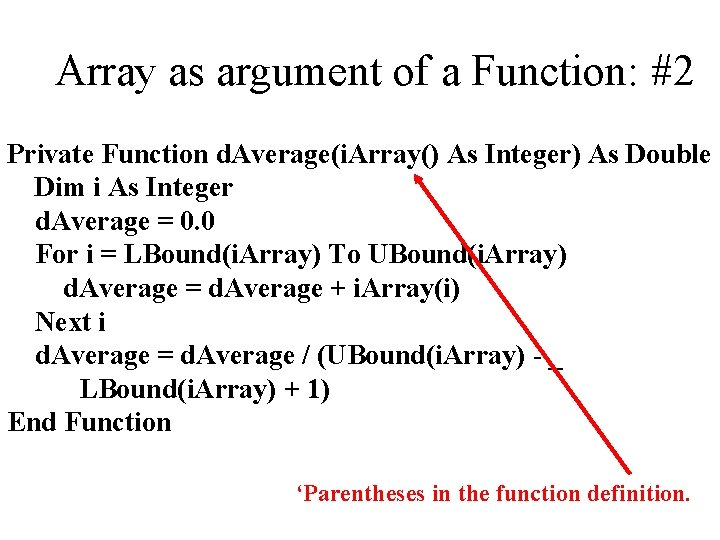Array as argument of a Function: #2 Private Function d. Average(i. Array() As Integer) As Double Dim i As Integer d. Average = 0. 0 For i = LBound(i. Array) To UBound(i. Array) d. Average = d. Average + i. Array(i) Next i d. Average = d. Average / (UBound(i. Array) - _ LBound(i. Array) + 1) End Function ‘Parentheses in the function definition.Array Bounds • The Lower Bound is the lowest index in an array (can be negative). – Index not value. • The Upper Bound is the highest index in an array (can be negative). • LBound(Array. Name) returns the lower bound of a variable array. • UBound(Array. Name) returns the upper bound of an variable array.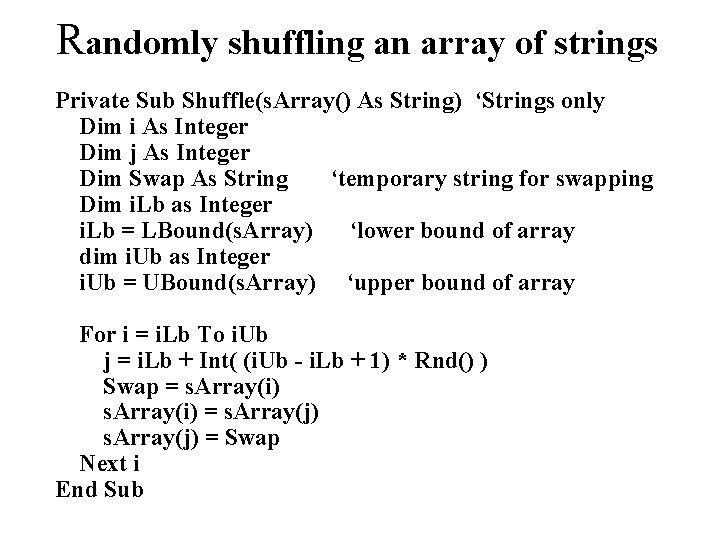Randomly shuffling an array of strings Private Sub Shuffle(s. Array() As String) ‘Strings only Dim i As Integer Dim j As Integer Dim Swap As String ‘temporary string for swapping Dim i. Lb as Integer i. Lb = LBound(s. Array) ‘lower bound of array dim i. Ub as Integer i. Ub = UBound(s. Array) ‘upper bound of array For i = i. Lb To i. Ub j = i. Lb + Int( (i. Ub - i. Lb + 1) * Rnd() ) Swap = s. Array(i) = s. Array(j) = Swap Next i End Sub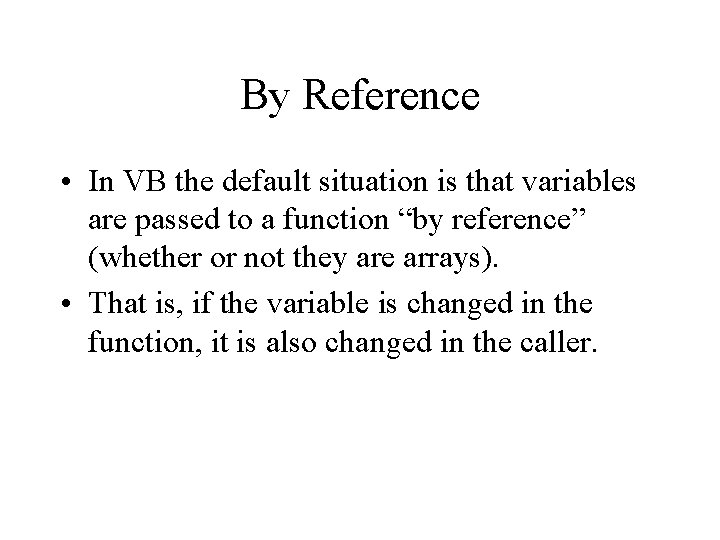By Reference • In VB the default situation is that variables are passed to a function “by reference” (whether or not they are arrays). • That is, if the variable is changed in the function, it is also changed in the caller.Control array • If a group of controls are – of the same type (e. g. all Command. Buttons) and – play a similar role (i. e. the code for them is nearly identical), it is convenient to make them an array • Section 7. 3 in Schneider (p. 338)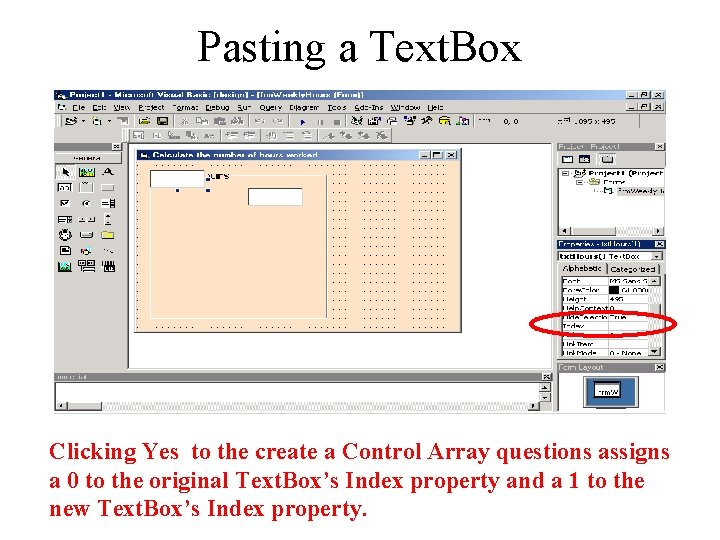Pasting a Text. Box Clicking Yes to the create a Control Array questions assigns a 0 to the original Text. Box’s Index property and a 1 to the new Text. Box’s Index property.Array of Text. BoxesCode for Array of Textboxes Option Explicit Private Sub cmd. Sum. Hours_Click() Dim i As Integer 'Counter Dim i. Total As Integer i. Total = 0 For i = 0 To txt. Hours. Ubound i. Total = i. Total + txt. Hours(i). Text Next i txt. Sum. Text = i. Total End Sub ‘For a control array Ubound is a property NOT function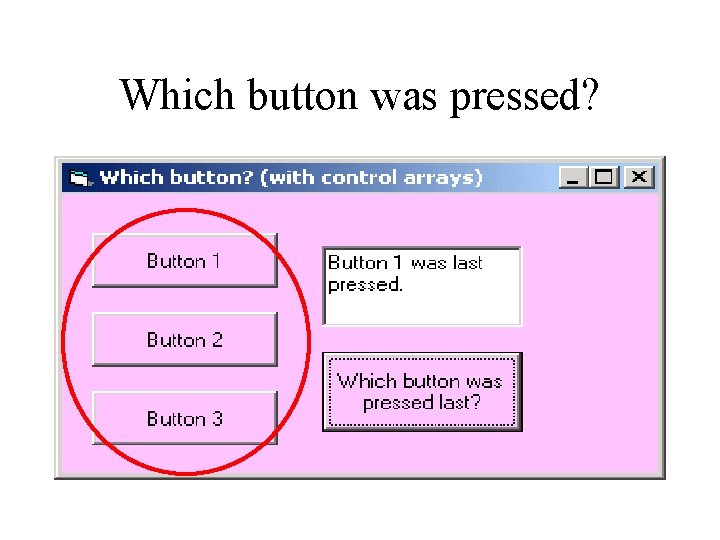Which button was pressed?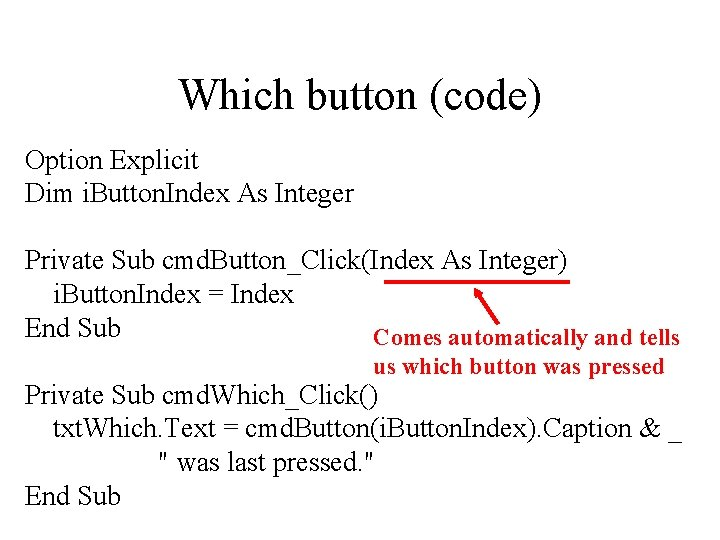Which button (code) Option Explicit Dim i. Button. Index As Integer Private Sub cmd. Button_Click(Index As Integer) i. Button. Index = Index End Sub Comes automatically and tells us which button was pressed Private Sub cmd. Which_Click() txt. Which. Text = cmd. Button(i. Button. Index). Caption & _ " was last pressed. " End Sub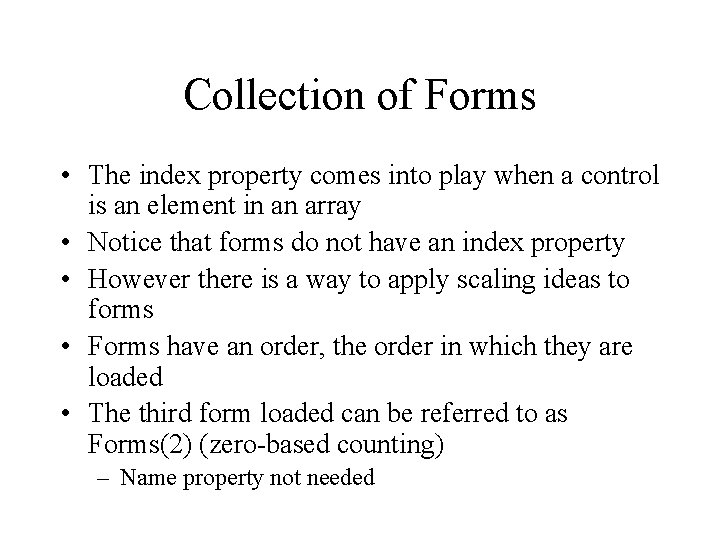Collection of Forms • The index property comes into play when a control is an element in an array • Notice that forms do not have an index property • However there is a way to apply scaling ideas to forms • Forms have an order, the order in which they are loaded • The third form loaded can be referred to as Forms(2) (zero-based counting) – Name property not needed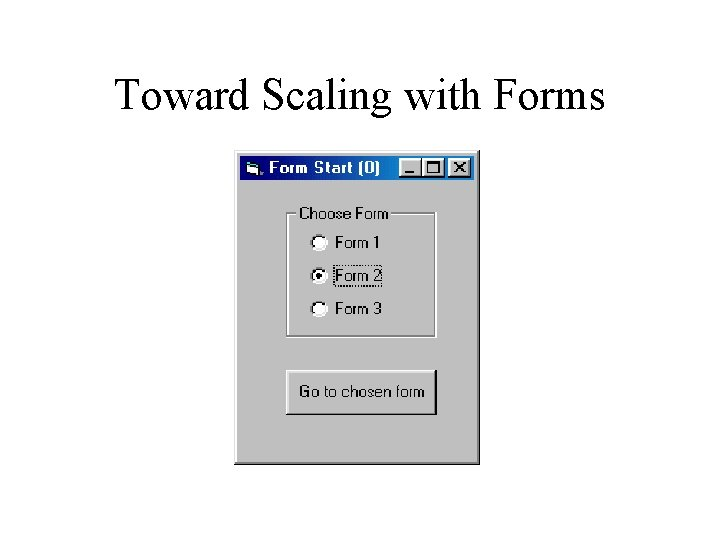Toward Scaling with Forms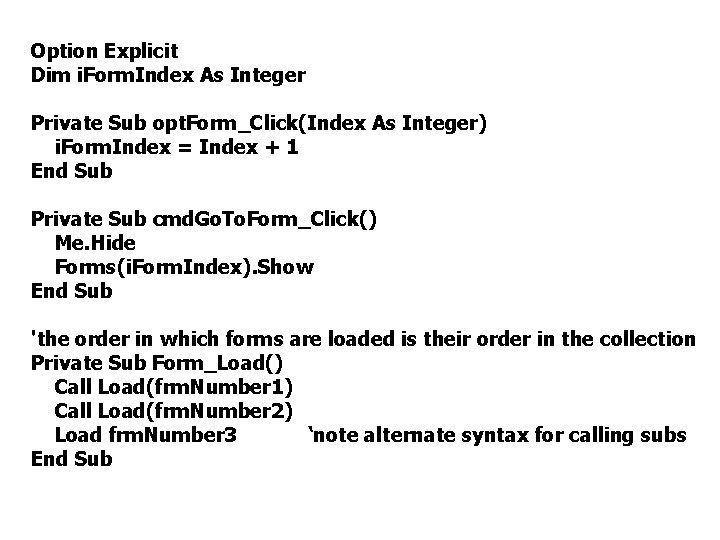Option Explicit Dim i. Form. Index As Integer Private Sub opt. Form_Click(Index As Integer) i. Form. Index = Index + 1 End Sub Private Sub cmd. Go. To. Form_Click() Me. Hide Forms(i. Form. Index). Show End Sub 'the order in which forms are loaded is their order in the collection Private Sub Form_Load() Call Load(frm. Number 1) Call Load(frm. Number 2) Load frm. Number 3 ‘note alternate syntax for calling subs End SubLISTBOXESFont Selection List. Box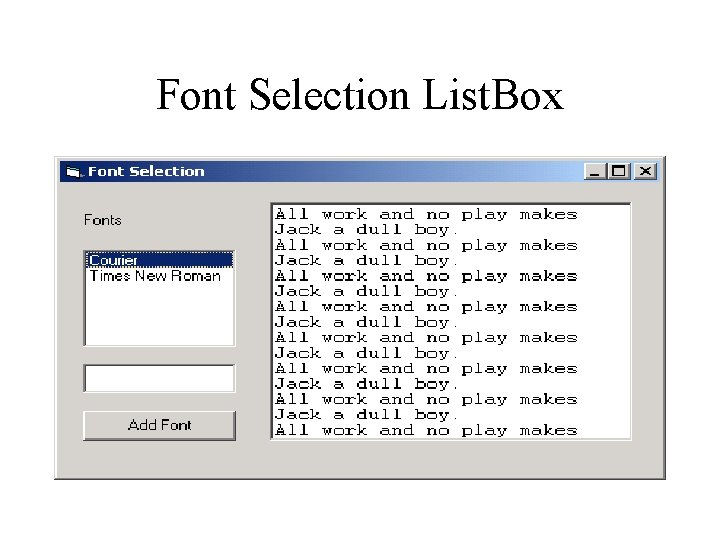Font Selection List. BoxFont Selection List. Box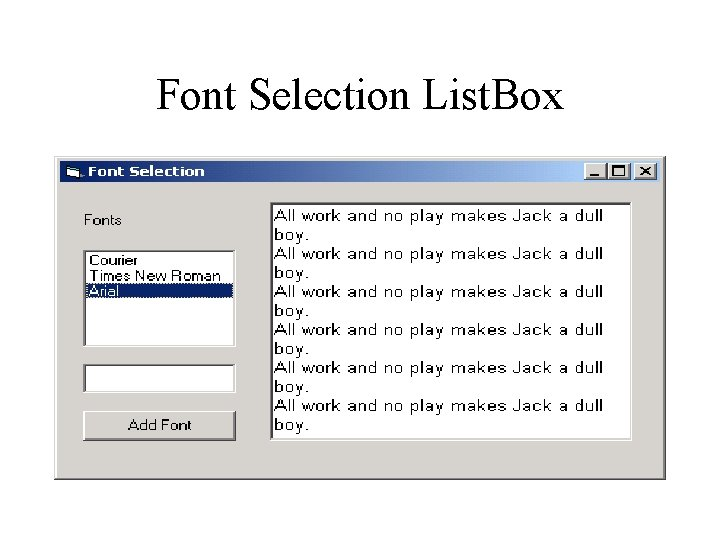Font Selection List. BoxFont Selection List. Box Option Explicit Has a click event Text of the currently highlighted item Private Sub lst. Font_Click() txt. Message. Font. Name = lst. Font. Text End Sub Private Sub cmd. Add. Font_Click() lst. Font. Add. Item (txt. New. Font. Text) txt. New. Font. Text = "" Method to add an End Sub item to the list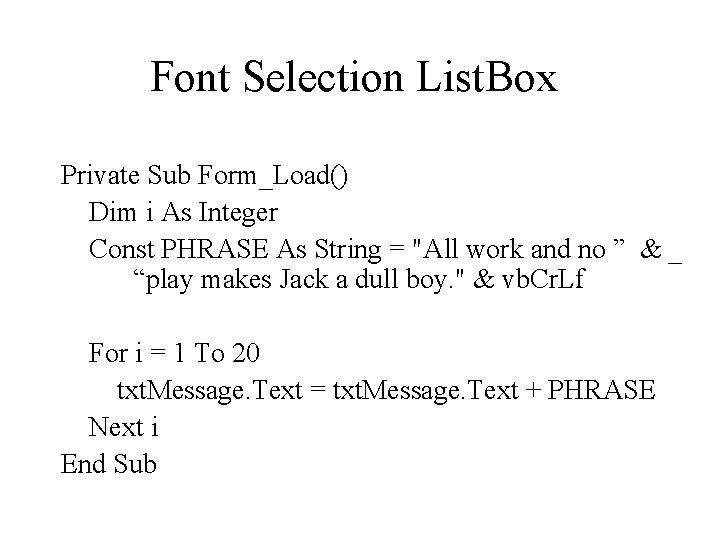Font Selection List. Box Private Sub Form_Load() Dim i As Integer Const PHRASE As String = "All work and no ” & _ “play makes Jack a dull boy. " & vb. Cr. Lf For i = 1 To 20 txt. Message. Text = txt. Message. Text + PHRASE Next i End SubChoose a Team List. Box Set listbox’s Multi. Select property to 1 in design time.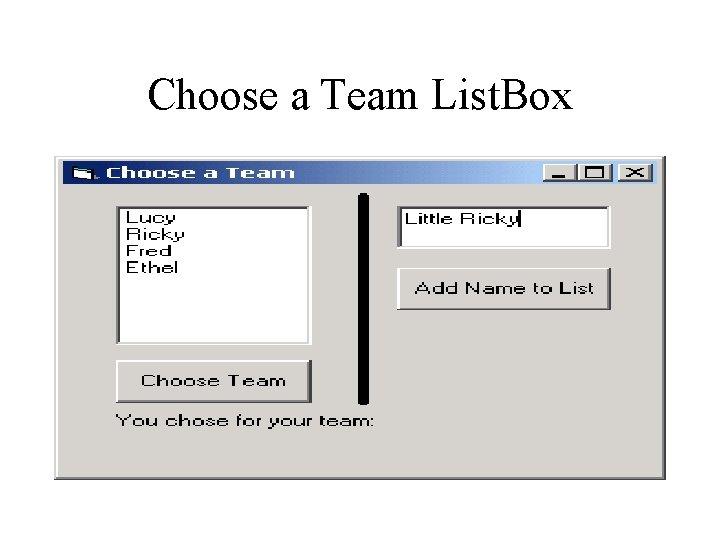Choose a Team List. BoxChoose a Team List. BoxChoose a Team List. Box Option Explicit Private Sub cmd. Add. Name_Click() lst. Names. Add. Item (txt. Name. Text) txt. Name. Text = "" End Sub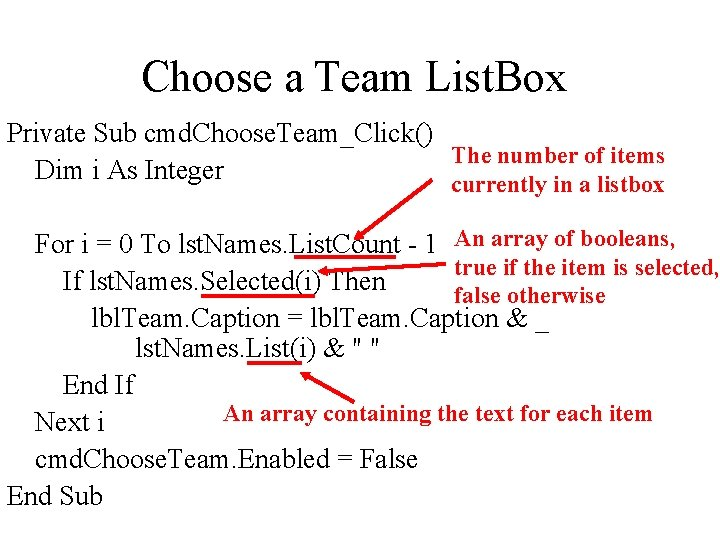Choose a Team List. Box Private Sub cmd. Choose. Team_Click() The number of items Dim i As Integer currently in a listbox For i = 0 To lst. Names. List. Count - 1 An array of booleans, true if the item is selected, If lst. Names. Selected(i) Then false otherwise lbl. Team. Caption = lbl. Team. Caption & _ lst. Names. List(i) & " " End If An array containing the text for each item Next i cmd. Choose. Team. Enabled = False End Sub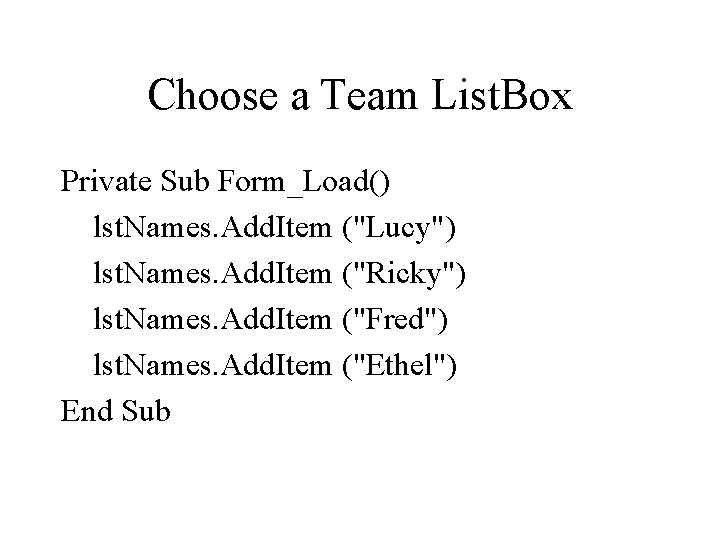Choose a Team List. Box Private Sub Form_Load() lst. Names. Add. Item ("Lucy") lst. Names. Add. Item ("Ricky") lst. Names. Add. Item ("Fred") lst. Names. Add. Item ("Ethel") End Sub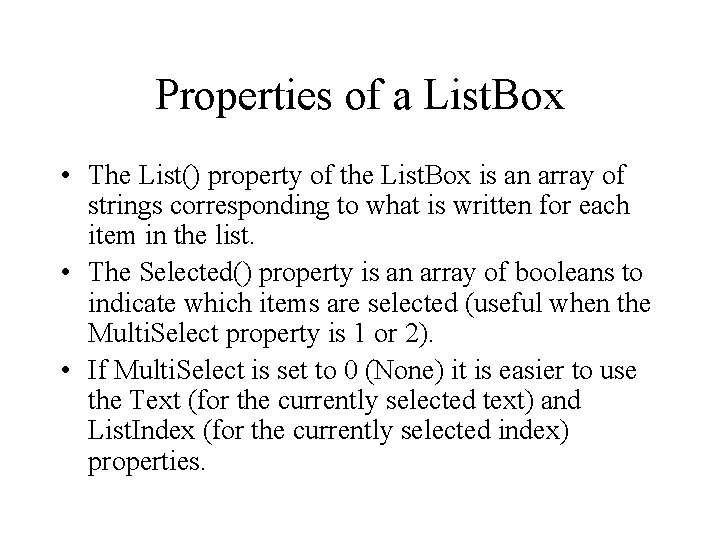Properties of a List. Box • The List() property of the List. Box is an array of strings corresponding to what is written for each item in the list. • The Selected() property is an array of booleans to indicate which items are selected (useful when the Multi. Select property is 1 or 2). • If Multi. Select is set to 0 (None) it is easier to use the Text (for the currently selected text) and List. Index (for the currently selected index) properties.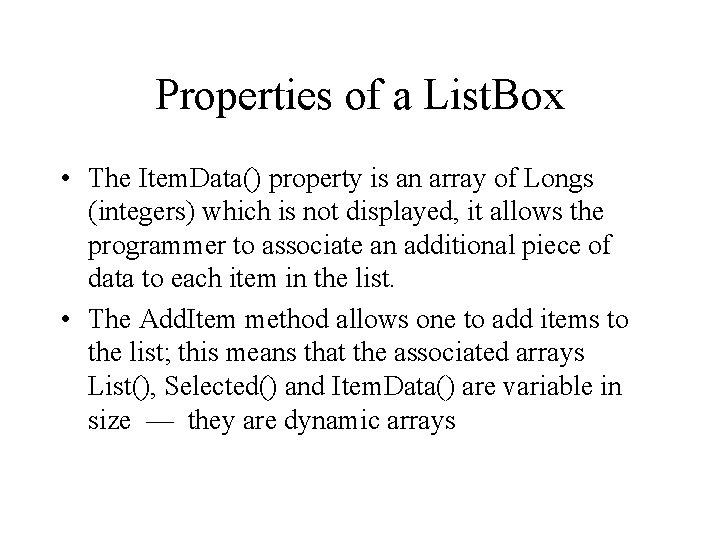Properties of a List. Box • The Item. Data() property is an array of Longs (integers) which is not displayed, it allows the programmer to associate an additional piece of data to each item in the list. • The Add. Item method allows one to add items to the list; this means that the associated arrays List(), Selected() and Item. Data() are variable in size — they are dynamic arrays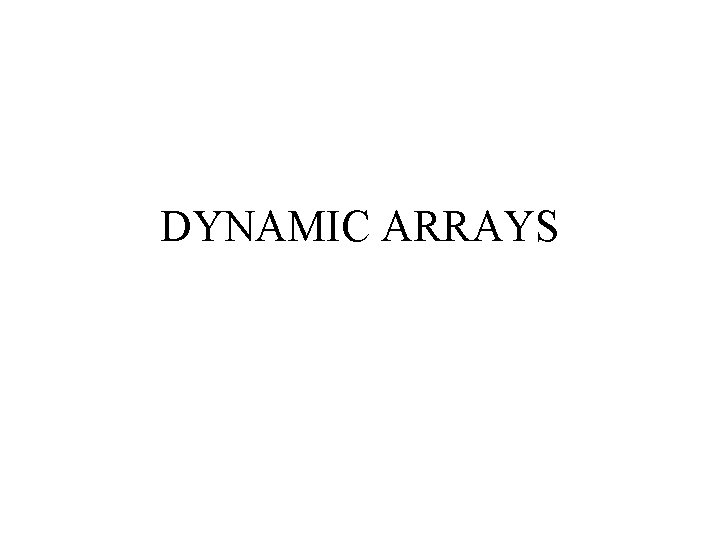DYNAMIC ARRAYS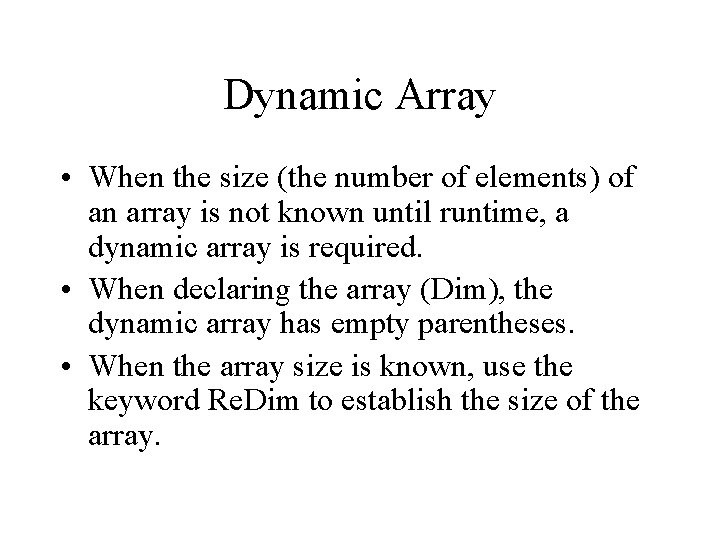Dynamic Array • When the size (the number of elements) of an array is not known until runtime, a dynamic array is required. • When declaring the array (Dim), the dynamic array has empty parentheses. • When the array size is known, use the keyword Re. Dim to establish the size of the array.Unknown number of gradesUnknown Number of Grades (Code) Option Explicit Dim i. Grades() As Integer Dim i. Number. Grades As Integer Don’t know size when declaring Private Sub cmd. Enter_Click() i. Number. Grades = txt. Grade. Number. Text - 1 Re. Dim i. Grades(i. Number. Grades) Now we know the Dim d. Ave As Double Dim i As Integer For i = LBound(i. Grades) To UBound(i. Grades) i. Grades(i) = Input. Box("Enter Grade " & i, "Grades") Next i d. Ave = d. Average(i. Grades) ‘Average function given before txt. Average. Text = d. Ave End Sub size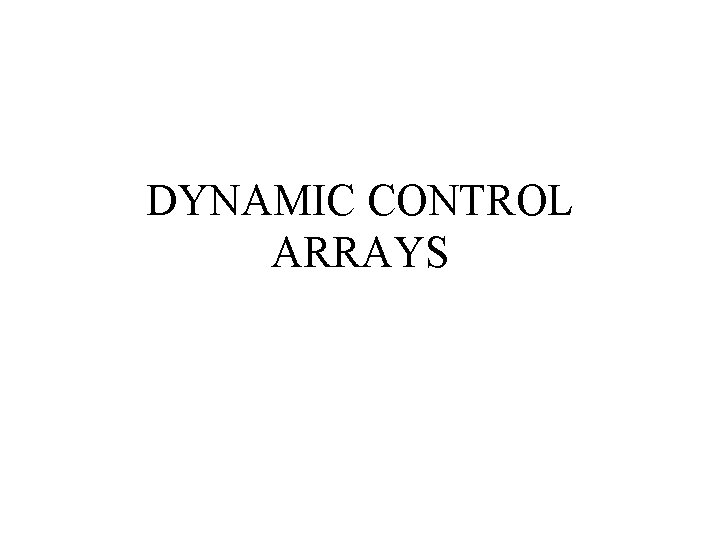DYNAMIC CONTROL ARRAYSDynamic Control Arrays • If there is unknown number of controls at design time, one needs a dynamic control array. • One drags and drops the first one onto the form, gives it an index of 0, and then adds others when the desired number of controls is known. • The problem with the dynamic control arrays is that they must be placed on the form, but where?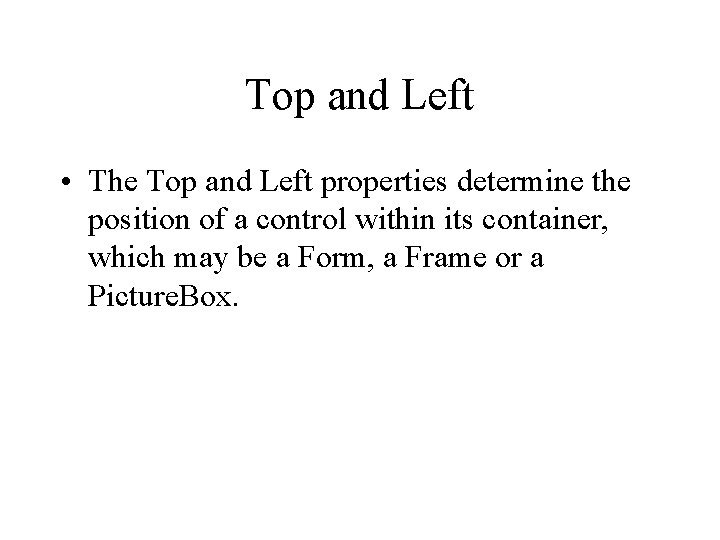Top and Left • The Top and Left properties determine the position of a control within its container, which may be a Form, a Frame or a Picture. Box.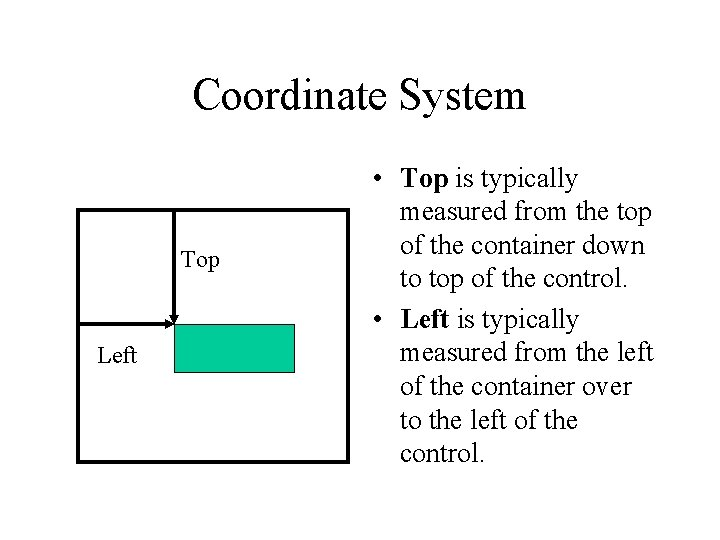Coordinate System Top Left • Top is typically measured from the top of the container down to top of the control. • Left is typically measured from the left of the container over to the left of the control.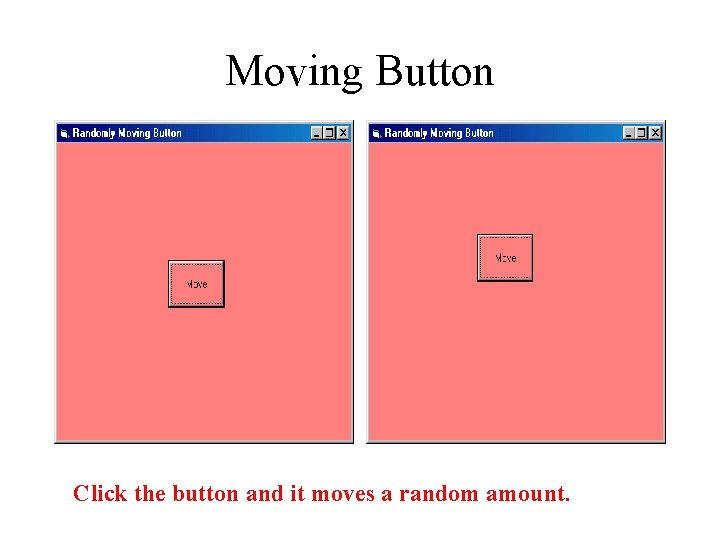Moving Button Click the button and it moves a random amount.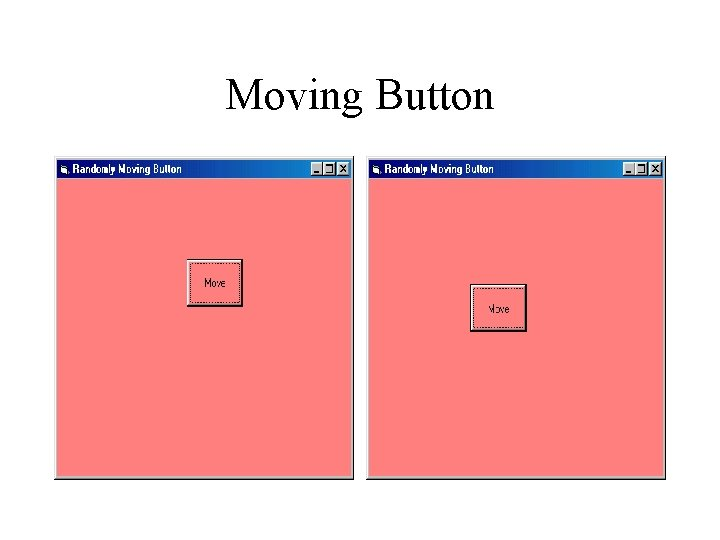Moving ButtonMoving Button (Code) Option Explicit Const MOVEAMOUNT AS Integer =1000 Private Sub cmd. Move_Click() cmd. Move. Top = cmd. Move. Top + MOVEAMOUNT _ * (1 - 2 * Rnd()) cmd. Move. Left = cmd. Move. Left + MOVEAMOUNT _ * (1 - 2 * Rnd()) If cmd. Move. Left < 0 Then cmd. Move. Left = 0 End If End Sub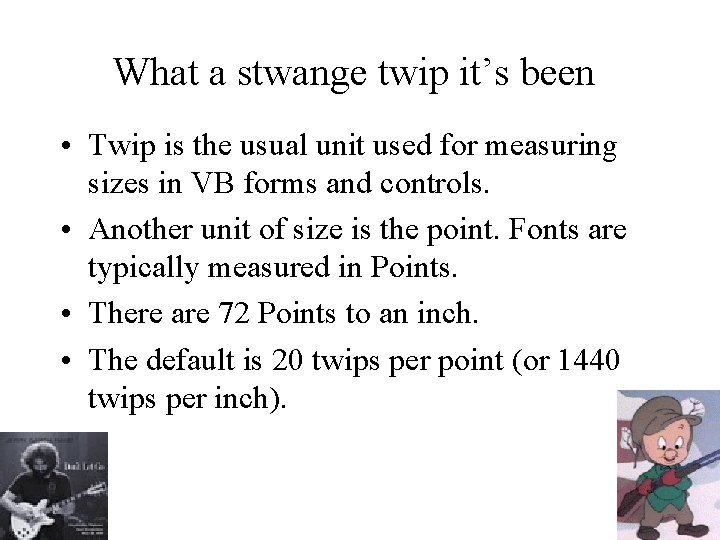What a stwange twip it’s been • Twip is the usual unit used for measuring sizes in VB forms and controls. • Another unit of size is the point. Fonts are typically measured in Points. • There are 72 Points to an inch. • The default is 20 twips per point (or 1440 twips per inch).Dynamic Control Array Generates a number of textboxes and labels determined at runtime.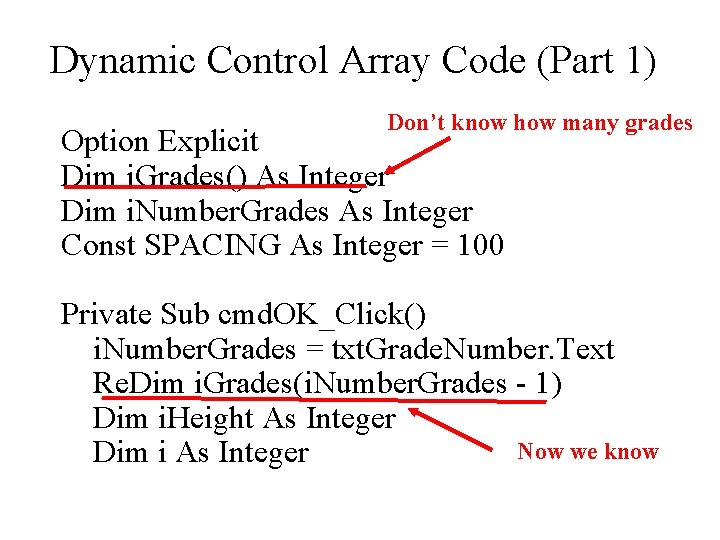Dynamic Control Array Code (Part 1) Don’t know how many grades Option Explicit Dim i. Grades() As Integer Dim i. Number. Grades As Integer Const SPACING As Integer = 100 Private Sub cmd. OK_Click() i. Number. Grades = txt. Grade. Number. Text Re. Dim i. Grades(i. Number. Grades - 1) Dim i. Height As Integer Now we know Dim i As Integer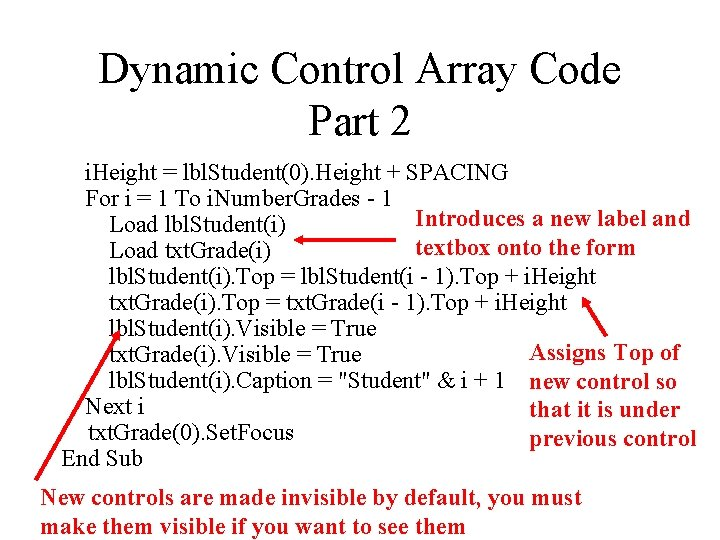Dynamic Control Array Code Part 2 i. Height = lbl. Student(0). Height + SPACING For i = 1 To i. Number. Grades - 1 Introduces a new label and Load lbl. Student(i) textbox onto the form Load txt. Grade(i) lbl. Student(i). Top = lbl. Student(i - 1). Top + i. Height txt. Grade(i). Top = txt. Grade(i - 1). Top + i. Height lbl. Student(i). Visible = True Assigns Top of txt. Grade(i). Visible = True lbl. Student(i). Caption = "Student" & i + 1 new control so Next i that it is under txt. Grade(0). Set. Focus previous control End Sub New controls are made invisible by default, you must make them visible if you want to see themDynamic Control Arrays • The first control in the array is placed on the form and given an index of 0 • When an element of a control array is loaded, it is given the same Top and Left properties as the original (same Height and Width too) • A more reasonable Top and Left must be provided, else the controls are superimposed – if you only see one control you either forgot to change left or top OR you did not make it visible.Dynamic Control Arrays • The newly loaded control has its Visible property set to False, so they must be made visible • If the program allows the size of the control array to be altered, then one might have to unload some of the controls – Unload them in reverse order • For i= lastcontrolnumber To 1 Step -1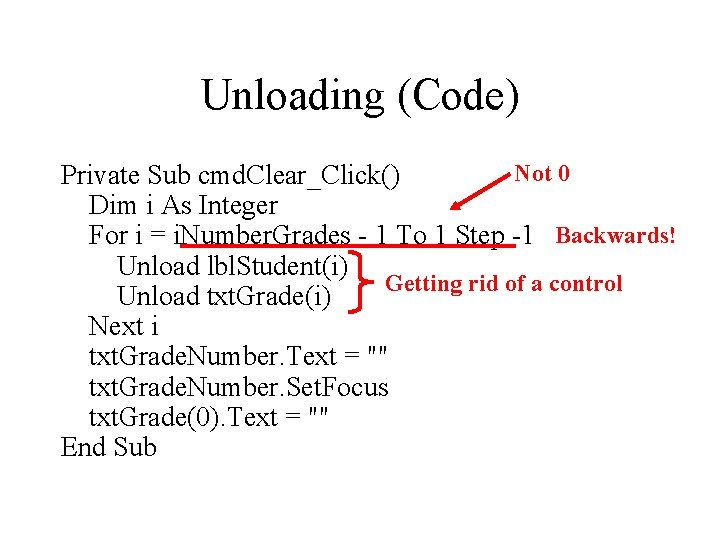Unloading (Code) Not 0 Private Sub cmd. Clear_Click() Dim i As Integer For i = i. Number. Grades - 1 To 1 Step -1 Backwards! Unload lbl. Student(i) Getting rid of a control Unload txt. Grade(i) Next i txt. Grade. Number. Text = "" txt. Grade. Number. Set. Focus txt. Grade(0). Text = "" End SubToward Rows and Columns: Integer Division • There are two ways to divide integers in VB – The first way results in a float (real number) • 15 / 4 3. 75 – The second way results in an integer (the whole number part of the above result) • 15 4 3 • Note VB uses a backward slash to indicate integer division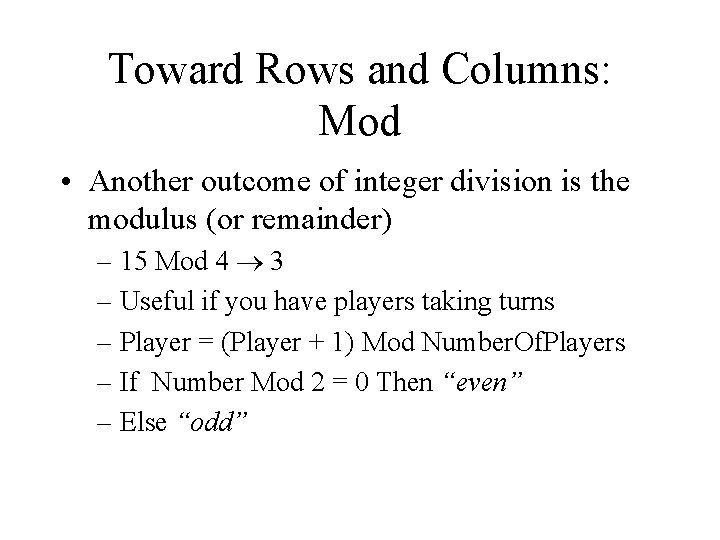Toward Rows and Columns: Mod • Another outcome of integer division is the modulus (or remainder) – 15 Mod 4 3 – Useful if you have players taking turns – Player = (Player + 1) Mod Number. Of. Players – If Number Mod 2 = 0 Then “even” – Else “odd”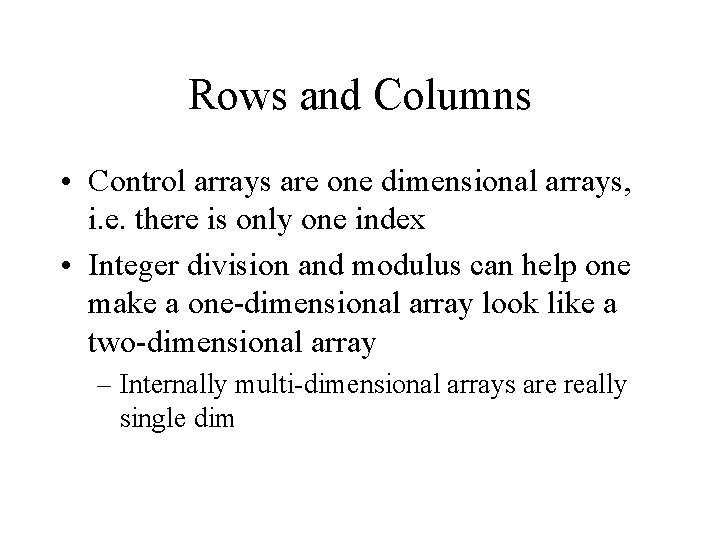Rows and Columns • Control arrays are one dimensional arrays, i. e. there is only one index • Integer division and modulus can help one make a one-dimensional array look like a two-dimensional array – Internally multi-dimensional arrays are really single dim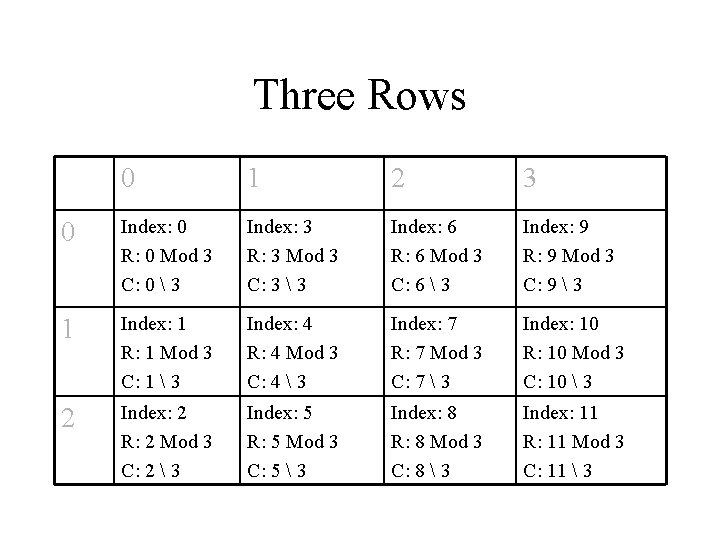Three Rows 0 1 2 3 0 Index: 0 R: 0 Mod 3 C: 0 3 Index: 3 R: 3 Mod 3 C: 3 3 Index: 6 R: 6 Mod 3 C: 6 3 Index: 9 R: 9 Mod 3 C: 9 3 1 Index: 1 R: 1 Mod 3 C: 1 3 Index: 4 R: 4 Mod 3 C: 4 3 Index: 7 R: 7 Mod 3 C: 7 3 Index: 10 R: 10 Mod 3 C: 10 3 2 Index: 2 R: 2 Mod 3 C: 2 3 Index: 5 R: 5 Mod 3 C: 5 3 Index: 8 R: 8 Mod 3 C: 8 3 Index: 11 R: 11 Mod 3 C: 11 3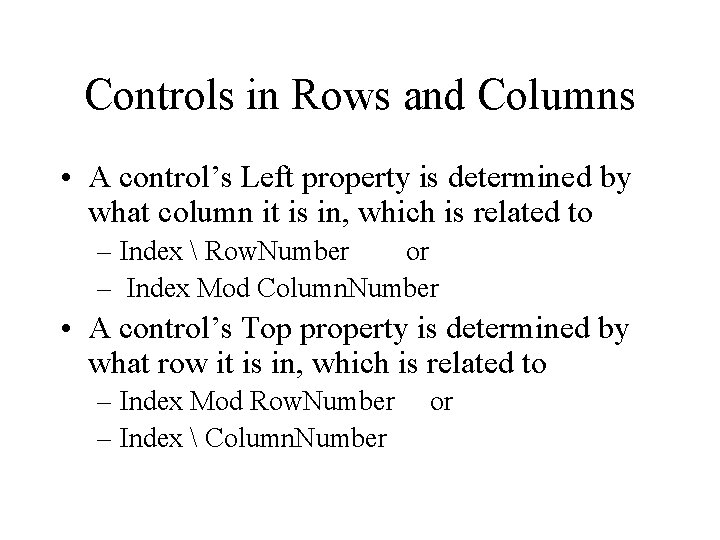Controls in Rows and Columns • A control’s Left property is determined by what column it is in, which is related to – Index Row. Number or – Index Mod Column. Number • A control’s Top property is determined by what row it is in, which is related to – Index Mod Row. Number – Index Column. Number orMarilyn and AndyMarilyn and Andy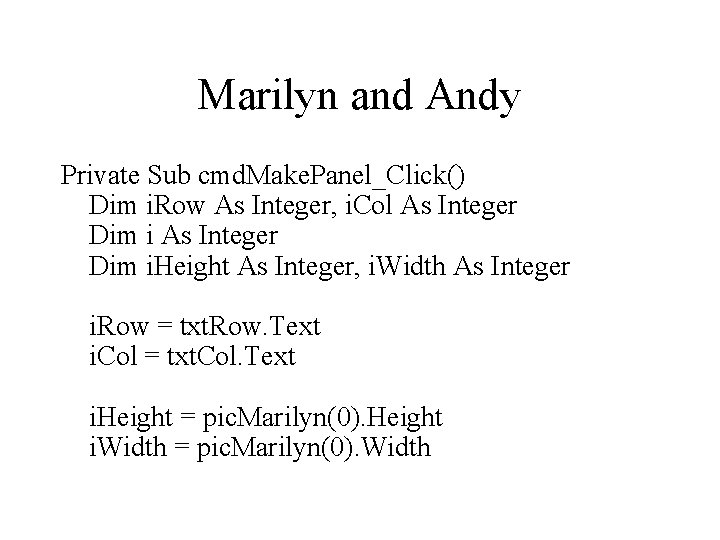Marilyn and Andy Private Sub cmd. Make. Panel_Click() Dim i. Row As Integer, i. Col As Integer Dim i. Height As Integer, i. Width As Integer i. Row = txt. Row. Text i. Col = txt. Col. Text i. Height = pic. Marilyn(0). Height i. Width = pic. Marilyn(0). Width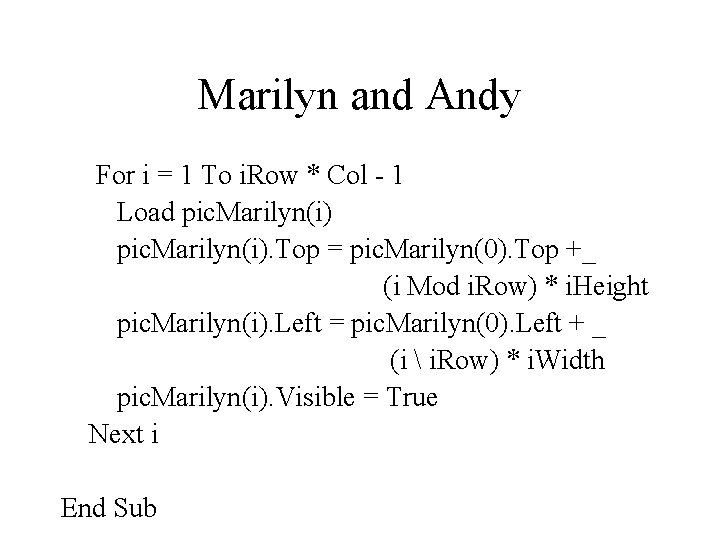Marilyn and Andy For i = 1 To i. Row * Col - 1 Load pic. Marilyn(i). Top = pic. Marilyn(0). Top +_ (i Mod i. Row) * i. Height pic. Marilyn(i). Left = pic. Marilyn(0). Left + _ (i i. Row) * i. Width pic. Marilyn(i). Visible = True Next i End Sub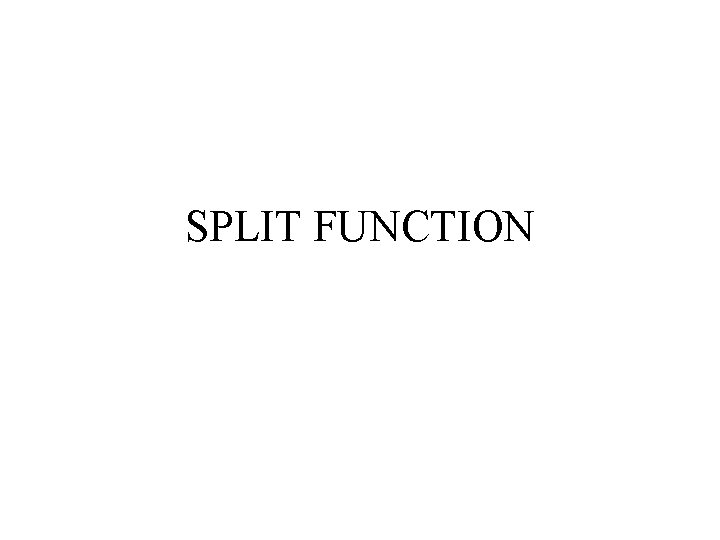SPLIT FUNCTION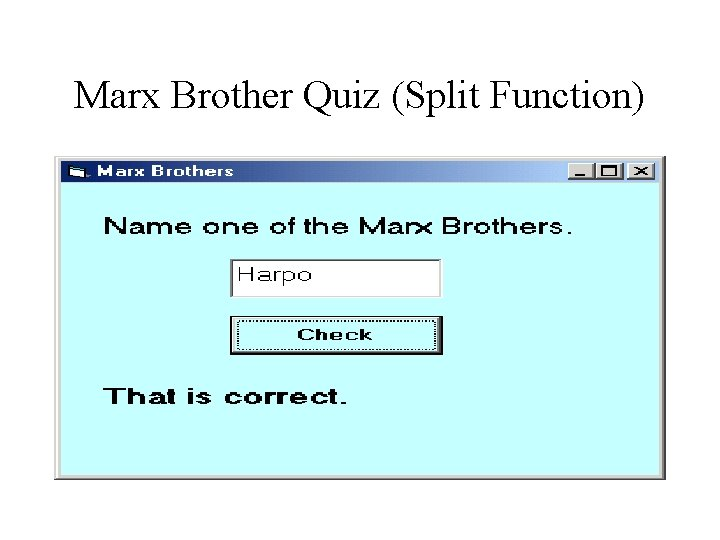Marx Brother Quiz (Split Function)Marx Brother Quiz (Split Function)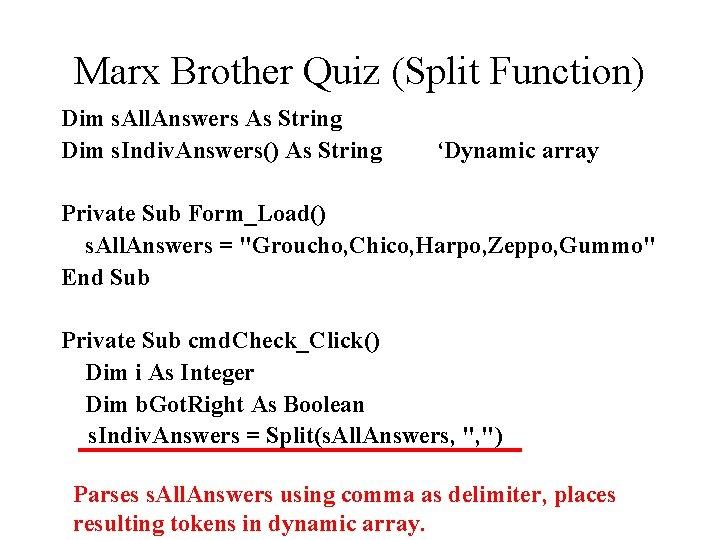Marx Brother Quiz (Split Function) Dim s. All. Answers As String Dim s. Indiv. Answers() As String ‘Dynamic array Private Sub Form_Load() s. All. Answers = "Groucho, Chico, Harpo, Zeppo, Gummo" End Sub Private Sub cmd. Check_Click() Dim i As Integer Dim b. Got. Right As Boolean s. Indiv. Answers = Split(s. All. Answers, ", ") Parses s. All. Answers using comma as delimiter, places resulting tokens in dynamic array.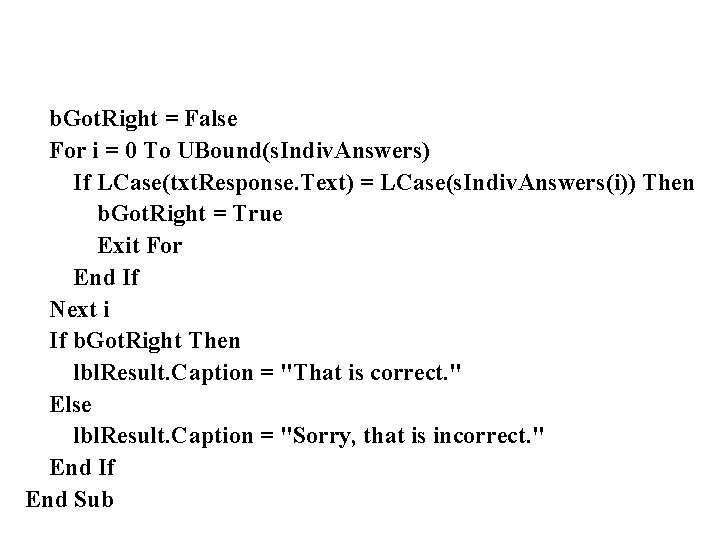b. Got. Right = False For i = 0 To UBound(s. Indiv. Answers) If LCase(txt. Response. Text) = LCase(s. Indiv. Answers(i)) Then b. Got. Right = True Exit For End If Next i If b. Got. Right Then lbl. Result. Caption = "That is correct. " Else lbl. Result. Caption = "Sorry, that is incorrect. " End If End Sub